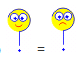Swindle? *****

User Rating:/ 1
PoorBestYES, you can swindleand prove theorems similar to this:

Swindle can be correctly used in many proofs, e.g.

Irreducibility of the sphere

Cantor–Bernstein–Schröder theorem ( Wiki EN )

...

Can you add the next one to the list?

A famous swindle (atributed to Samuel Eilenberg and Barry Mazur, Wiki EN) uses a foolish

1 = 1 + (−1 + 1) + (−1 + 1) + ... = 1 − 1 + 1 − 1 + ... = (1 − 1) + (1 − 1) + ... = 0

identity idea to prove various correct results in a true correct way. Read great EXPO and a DISCUSSION on Terrence Tao blog ( EN ).=+ (+) + (+) + ... =

=++++ ... =++++ ... =

=+ (+) + (+) + ... =